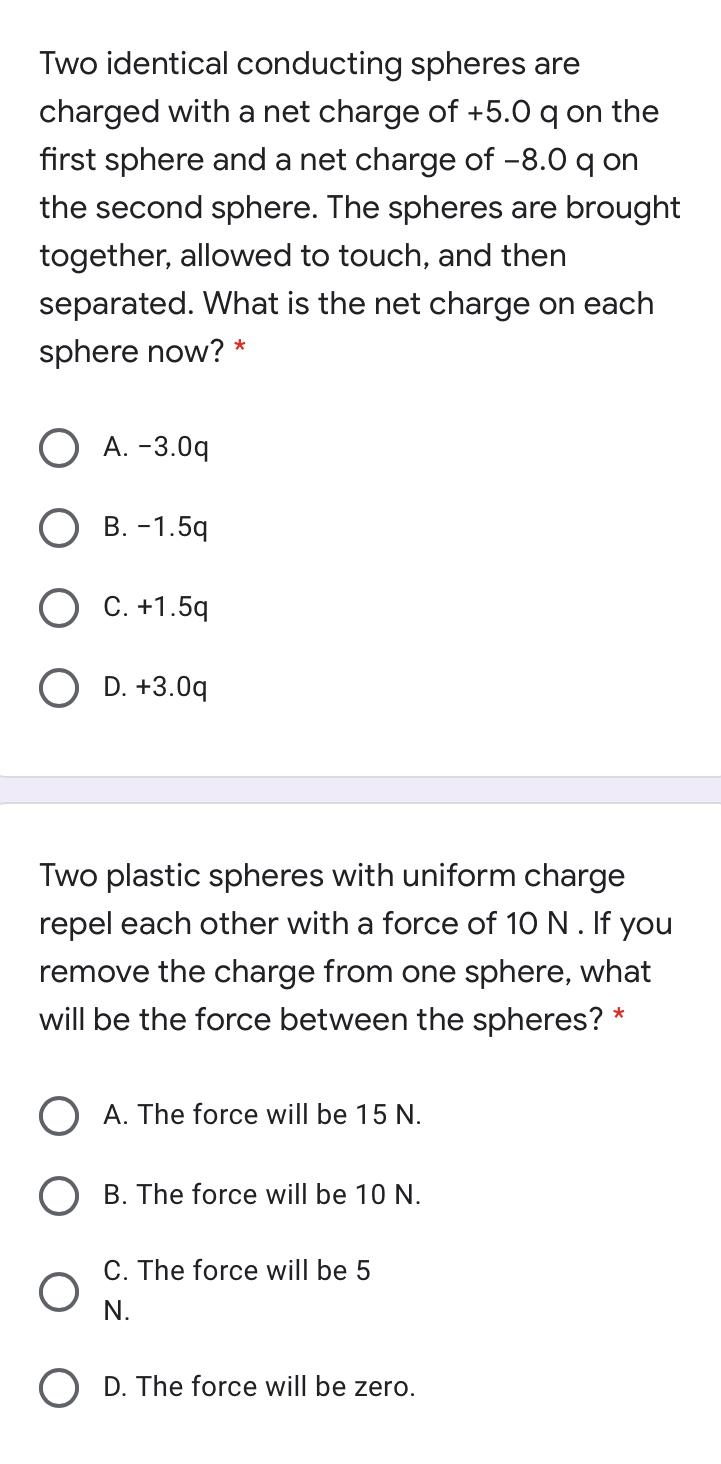Question:

# Two identical conducting spheres are charged with a net charge of +5.0 qon the first sphere and a net charge of -8.0 qon the secTwo identical conducting spheres are charged with a net charge of +5.0 qon the first sphere and a net charge of -8.0 qon the second sphere. The spheres are brought together, allowed to touch, and then separated. What is the net charge on each sphere now? * A. -3.09 O B. -1.59 O C. +1.59 O D. +3.00 Two plastic spheres with uniform charge repel each other with a force of 10 N. If you remove the charge from one sphere, what will be the force between the spheres? * O A. The force will be 15 N. O B. The force will be 10 N. C. The force will be 5 N. D. The force will be zero.